# Selina solutions for Concise Maths Class 10 ICSE chapter 4 - Linear Inequations (In one variable) [Latest edition]

#### Chapters## Chapter 4: Linear Inequations (In one variable)

Exercise 4 (A)Exercise 4 (B)
Exercise 4 (A) [Page 44]

### Selina solutions for Concise Maths Class 10 ICSE Chapter 4 Linear Inequations (In one variable) Exercise 4 (A) [Page 44]

Exercise 4 (A) | Q 1.1 | Page 44

State true or false

x < -y => -x > y

• True

• False

Exercise 4 (A) | Q 1.2 | Page 44

State true or false

-5x >= 15 => x >= -3

• True

• False

Exercise 4 (A) | Q 1.3 | Page 44

State true or false

2x <= -7 => (2x)/(-4) >= (-7)/(-4)

• True

• False

Exercise 4 (A) | Q 1.4 | Page 44

State true or false

7 > 5 => 1/7 < 1/5

• True

• False

Exercise 4 (A) | Q 2.1 | Page 44

State, whether the following statements are true or false:

a < b, then a – c < b – c

• True

• False

Exercise 4 (A) | Q 2.2 | Page 44

State, whether the following statements are true or false:

If a > b, then a + c > b + c

• True

• False

Exercise 4 (A) | Q 2.3 | Page 44

State, whether the following statements are true or false:

IF a < b, then ac < bc

• True

• False

Exercise 4 (A) | Q 2.4 | Page 44

State, whether the following statements are true or false:

if a > b then a/c < b/c

• True

• False

Exercise 4 (A) | Q 2.5 | Page 44

State, whether the following statements are true or false:

If a – c > b – d, then a + d > b + c

• True

• False

Exercise 4 (A) | Q 2.6 | Page 44

State, whether the following statements are true or false:

If a < b, and c > 0, then a – c < b – c
Where a, b, c and d are real numbers and c ≠ 0.

• True

• False

Exercise 4 (A) | Q 3.1 | Page 44

If x ∈ N, find the solution set of inequations.

5x + 3 ≤ 2x + 18

Exercise 4 (A) | Q 3.2 | Page 44

If x ∈ N, find the solution set of inequations.

3x – 2 < 19 – 4x

Exercise 4 (A) | Q 4.1 | Page 44

If the replacement set is the set of whole numbers, solve :

x + 7 <= 11

Exercise 4 (A) | Q 4.2 | Page 44

If the replacement set is the set of whole numbers solve:

3x - 1 > 8

Exercise 4 (A) | Q 4.3 | Page 44

If the replacement set is the set of whole numbers solve

8 - x > 5

Exercise 4 (A) | Q 4.4 | Page 44

If the replacement set is the set of whole numbers solve

7 - 3x >= - 1/2

Exercise 4 (A) | Q 4.5 | Page 44

If the replacement set is the set of whole numbers solve

x  - 3/2 < 3/2 - x

Exercise 4 (A) | Q 4.6 | Page 44

If the replacement set is the set of whole numbers solve

18<= 3"x" - 2

Exercise 4 (A) | Q 5 | Page 44

Solve the inequation:

3 – 2x ≥ x – 12 given that x ∈ N.

Exercise 4 (A) | Q 6 | Page 44

If 25 – 4x ≤ 16, find:

(1) the smallest value of x, when x is a real number,

(2) the smallest value of x, when x is an integer.

Exercise 4 (A) | Q 7.1 | Page 44

If the replacement set is the set of real numbers solve

-4x >= - 16

Exercise 4 (A) | Q 7.2 | Page 44

If the replacement set is the set of real numbers solve

8 - 3x  <= 20

Exercise 4 (A) | Q 7.3 | Page 44

If the replacement set is the set of real numbers solve

5 + x/4 > x/5 + 9

Exercise 4 (A) | Q 7.4 | Page 44

If the replacement set is the set of real numbers solve

("x" + 3)/8 < ("x" - 3)/5

Exercise 4 (A) | Q 8 | Page 44

Find the smallest value of x for which 5 - 2"x" < 5 1/2 - 5/3"x" where x  is interger

Exercise 4 (A) | Q 9 | Page 44

Find the largest value of x for which 2(x – 1) ≤ 9 – x and x ∈ W.

Exercise 4 (A) | Q 10 | Page 44

Solve the inequation 12 + 1 5/6 xx ≤ 5 + 3"x" and "x"  in "R"

Exercise 4 (A) | Q 11 | Page 44

Given x ∈ {integers}, find the solution set of:

-5 ≤ 2x – 3 < x + 2

Exercise 4 (A) | Q 12 | Page 44

Given x ∈ {whole numbers}, find the solution set of: -1 ≤ 3 + 4x < 23

Exercise 4 (B) [Pages 49 - 50]

### Selina solutions for Concise Maths Class 10 ICSE Chapter 4 Linear Inequations (In one variable) Exercise 4 (B) [Pages 49 - 50]

Exercise 4 (B) | Q 1.1 | Page 49

Represent the following inequalities on real number lines

2x - 1 < 5

Exercise 4 (B) | Q 1.2 | Page 49

Represent the following inequalities on real number lines

3x + 1 >= -5

Exercise 4 (B) | Q 1.3 | Page 49

Represent the following inequalities on real number lines

2(2x- 3) <= 6

Exercise 4 (B) | Q 1.4 | Page 49

Represent the following inequalities on real number lines

-4 < x < 4

Exercise 4 (B) | Q 1.5 | Page 49

Represent the following inequalities on real number lines

-2 <= x  < 5

Exercise 4 (B) | Q 1.6 | Page 49

Represent the following inequalities on real number lines

8 >= x > -3

Exercise 4 (B) | Q 1.7 | Page 49

Represent the following in-equalities on real number line :

−5 < × ≤ −1

Exercise 4 (B) | Q 2.1 | Page 49

For graph given write an inequation taking x as the variable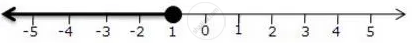Exercise 4 (B) | Q 2.2 | Page 49

For graph given write an inequation taking x as the variable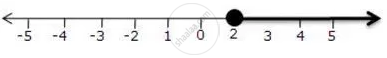Exercise 4 (B) | Q 2.3 | Page 49

For graph given write an inequation taking x as the variable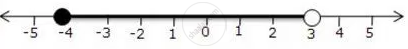Exercise 4 (B) | Q 2.4 | Page 49

For graph given write an inequation taking x as the variable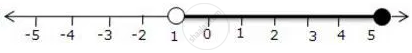Exercise 4 (B) | Q 3.1 | Page 49

For the given inequations graph the solution set on the real number line

-4 < 3x - 1 < 8

Exercise 4 (B) | Q 3.2 | Page 49

For the given inequations graph the solution set on the real number line

x - 1 < 3 -  x <= 5

Exercise 4 (B) | Q 4.1 | Page 49

Represent the solution of the given inequalities on the real number line

4x - 1 > x + 11

Exercise 4 (B) | Q 4.2 | Page 49

Represent the solution of the given inequalities on the real number line

7 - x <= 2 - 6x

Exercise 4 (B) | Q 4.3 | Page 49

Represent the solution of the given inequalities on the real number line

x + 3 <= 2x + 9

Exercise 4 (B) | Q 4.4 | Page 49

Represent the solution of the given inequalities on the real number line

2 - 3x > 7 - 5x

Exercise 4 (B) | Q 4.5 | Page 49

Represent the solution of the given inequalities on the real number line

1 + x >= 5x - 11

Exercise 4 (B) | Q 4.6 | Page 49

Represent the solution of the given inequalities on the real number line

(2x + 5)/3 > 3x - 3

Exercise 4 (B) | Q 5 | Page 49

x ∈ {real numbers} and -1 < 3 – 2x ≤ 7, evaluate x and represent it on a number line.

Exercise 4 (B) | Q 6 | Page 49

List the elements of the solution set of the inequation

-3 < x – 2 ≤ 9 – 2x; x ∈ N.

Exercise 4 (B) | Q 7 | Page 49

Find the range of values of x which satisfies

-2 2/3 <= x + 1/3 < 3 1/3; x in R

Graph these values of x on the number line.

Exercise 4 (B) | Q 8 | Page 49

Find the values of x which satisfy the inequation

-2 <= 1/2 - (2x)/3 < 1 5/6; x ∈ N

Graph the solution on the number line

Exercise 4 (B) | Q 9 | Page 49

Given x ∈ {real numbers}, find the range of values of x for which -5 ≤ 2x – 3 < x + 2 and represent it on a number line.

Exercise 4 (B) | Q 10 | Page 49

If 5x – 3 ≤ 5 + 3x ≤ 4x + 2, express it as a ≤ x ≤ b and then state the values of a and b.

Exercise 4 (B) | Q 11 | Page 49

Solve the following inequation and graph the solution set on the number line:
2x – 3 < x + 2 ≤ 3x + 5, x ∈ R.

Exercise 4 (B) | Q 12.1 | Page 49

Solve and graph the solution set of:

2x – 9 < 7 and 3x + 9 ≤ 25, x ∈ R

Exercise 4 (B) | Q 12.2 | Page 49

Solve and graph the solution set of:

2x – 9 ≤ 7 and 3x + 9 > 25, x ∈ I

Exercise 4 (B) | Q 12.3 | Page 49

Solve and graph the solution set of:

x + 5 ≥ 4(x - 1) and 3 - 2x < -7 ; x ∈ R .

Exercise 4 (B) | Q 13.1 | Page 49

Solve and graph the solution set of:

3x – 2 > 19 or 3 – 2x ≥ -7, x ∈ R

Exercise 4 (B) | Q 13.2 | Page 49

Solve and graph the solution set of:

5 > p – 1 > 2 or 7 ≤ 2p – 1 ≤ 17, p ∈ R

Exercise 4 (B) | Q 14 | Page 49

The diagram represents two inequations A and B on real number lines: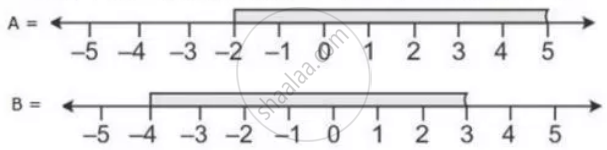1) Write down A and B in set builder notation/

2) Represent A ∪ B and A ∩ B' on two different number lines

Exercise 4 (B) | Q 15.1 | Page 49

Use the real number line to find the range of values of x for which:

x > 3 and 0 < x < 6

Exercise 4 (B) | Q 15.2 | Page 49

Use the real number line to find the range of values of x for which:

x < 0 and -3 ≤ x < 1

Exercise 4 (B) | Q 15.3 | Page 49

Use the real number line to find the range of values of x for which:

-1 < x ≤ 6 and -2 ≤ x ≤ 3

Exercise 4 (B) | Q 16 | Page 49

Illustrate the set {x: -3 ≤ x < 0 or x > 2, x ∈ R} on the real number line.

Exercise 4 (B) | Q 17.1 | Page 50

Given A = {x: -1 < x ≤ 5, x ∈ R} and B = {x: -4 ≤ x < 3, x ∈ R}

Represent on different number lines:

A ∩ B

Exercise 4 (B) | Q 17.2 | Page 50

Given A = {x: -1 < x ≤ 5, x ∈ R} and B = {x: -4 ≤ x < 3, x ∈ R}
Represent on different number lines:

A' ∩ B

Exercise 4 (B) | Q 17.3 | Page 50

Given A = {x: -1 < x ≤ 5, x ∈ R} and B = {x: -4 ≤ x < 3, x ∈ R}
Represent on different number lines:

A – B

Exercise 4 (B) | Q 18 | Page 50

P is the solution set of 7x – 2 > 4x + 1 and Q is the solution set of 9x – 45 ≥ 5(x – 5); where x ∈ R. Represent:

1) P ∩ Q

2) P – Q

3) P ∩ Q’

on the different number of lines.

Exercise 4 (B) | Q 19 | Page 50

If P = {x: 7x — 4 > 5x + 2, x ∈ R} and Q = {x: x — 19 ≥ 1 — 3x, x ∈ R}, find the range of set P ∩ Q and represent it on a number line.

Exercise 4 (B) | Q 20 | Page 50

Find the range of values of x which satisfy:

- 1/3 <= x/2 + 1 2/3 < 5 1/6

The graph in each of the following cases the values of x on the different real number lines:

1) x ∈ W

2) x ∈ Z

3) x ∈ R

Exercise 4 (B) | Q 21 | Page 50

Given: A = {x: -8 < 5x + 2 ≤ 17, x ∈ I}, B = {x: -2 ≤ 7 + 3x < 17, x ∈ R}
Where R = {real numbers} and I = {integers}. Represent A and B on two different number lines. Write down the elements of A ∩ B.

Exercise 4 (B) | Q 22 | Page 50

Solve the following inequation and represent the solution set on the number line 2x – 5 ≤ 5x +4 < 11, where x ∈ I

Exercise 4 (B) | Q 23 | Page 50

Given that x ∈ I. solve the inequation and graph the solution on the number line:

3 >= (x - 4)/2 + x/3 >= 2

Exercise 4 (B) | Q 24 | Page 50

Given:
A = {x: 11x – 5 > 7x + 3, x ∈ R} and
B = {x: 18x – 9 ≥ 15 + 12x, x ∈ R}.
Find the range of set A ∩ B and represent it on the number line.

Exercise 4 (B) | Q 25 | Page 50

Find the set of values of x satisfying

7x + 3 >= 3x - 5 and x/4 - 5 <= 5/4 - x where x ∈ N

Exercise 4 (B) | Q 26.1 | Page 50

Solve

x/2 + 5 <= x/3 +6 where x is a positive odd integer.

Exercise 4 (B) | Q 26.2 | Page 50

Solve

(2x + 3)/3 >= (3x - 1)/4 where x is a positive even integer.

Exercise 4 (B) | Q 27 | Page 50

Solve the inequation

-2 1/2 + 2x <= (4x)/5 <= 4/3 + 2x , x ∈ W.

Graph the solution set on the number line.

Exercise 4 (B) | Q 28 | Page 50

Find three consecutive largest positive integers such that the sum of one-third of first, one-fourth of second and one-fifth of third is almost 20.

Exercise 4 (B) | Q 29 | Page 50

Solve the given inequation and graph the solution on the number line.
2y – 3 < y + 1 ≤ 4y + 7, y ∈ R

Exercise 4 (B) | Q 30 | Page 50

Solve the inequation:
3z – 5 ≤ z + 3 < 5z – 9, z ∈ R.
Graph the solution set on the number line

Exercise 4 (B) | Q 31 | Page 50

Solve the following inequation and represent the solution set on the number line

-3 < -1/2 - (2x)/3 ≤ 5/6, x in R

Exercise 4 (B) | Q 32 | Page 50

Solve the following inequation and represent the solution set on the number line

4x - 19 < (3x)/5 - 2 <= (-2)/5 + x, x ∈ R

Exercise 4 (B) | Q 33 | Page 50

Solve the following in equation, write the solution set and represent it on the number line:

-"x"/3≤ "x"/2 -1 1/3<1/6, "x" in "R"

Exercise 4 (B) | Q 34 | Page 50

Find the values of x, which satify the inequation

-25/6 < 1/2 - (2"x")/3 ≤ 2, "x" in "W"

Graph the solution set on the number line.

Exercise 4 (B) | Q 35 | Page 50

Solve the following in equation and write the solution set:

13x - 5 < 15x + 4 < 7x + 12, x ∈ R

Represent the solution on a real number line.

Exercise 4 (B) | Q 36 | Page 50

Solve the following inequation, write the solution set and represent it on the number line.

-3 (x - 7) ≥ 15 - 7x > ("x" + 1)/3,"x" in "R"

Exercise 4 (B) | Q 37 | Page 50

Solve the following inequation and represent the solution set on a number line.

-8 1/2 < -1/2 - 4x ≤ 71/2, "x" in "I"

## Chapter 4: Linear Inequations (In one variable)

Exercise 4 (A)Exercise 4 (B)## Selina solutions for Concise Maths Class 10 ICSE chapter 4 - Linear Inequations (In one variable)

Selina solutions for Concise Maths Class 10 ICSE chapter 4 (Linear Inequations (In one variable)) include all questions with solution and detail explanation. This will clear students doubts about any question and improve application skills while preparing for board exams. The detailed, step-by-step solutions will help you understand the concepts better and clear your confusions, if any. Shaalaa.com has the CISCE Concise Maths Class 10 ICSE solutions in a manner that help students grasp basic concepts better and faster.

Further, we at Shaalaa.com provide such solutions so that students can prepare for written exams. Selina textbook solutions can be a core help for self-study and acts as a perfect self-help guidance for students.

Concepts covered in Concise Maths Class 10 ICSE chapter 4 Linear Inequations (In one variable) are Linear Inequations in One Variable, Solving Algebraically and Writing the Solution in Set Notation Form, Representation of Solution on the Number Line.

Using Selina Class 10 solutions Linear Inequations (In one variable) exercise by students are an easy way to prepare for the exams, as they involve solutions arranged chapter-wise also page wise. The questions involved in Selina Solutions are important questions that can be asked in the final exam. Maximum students of CISCE Class 10 prefer Selina Textbook Solutions to score more in exam.

Get the free view of chapter 4 Linear Inequations (In one variable) Class 10 extra questions for Concise Maths Class 10 ICSE and can use Shaalaa.com to keep it handy for your exam preparation